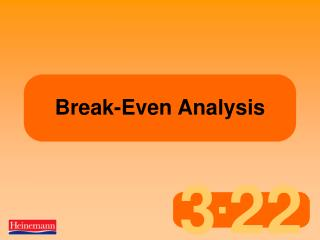DownloadDownload PresentationBreak-Even Analysis

# Break-Even Analysis

Télécharger la présentation## Break-Even Analysis

- - - - - - - - - - - - - - - - - - - - - - - - - - - E N D - - - - - - - - - - - - - - - - - - - - - - - - - - -
##### Presentation Transcript

1. Break-Even Analysis

2. Terminology • Sales Revenue / Income – The amount of money a company takes from selling goods or services. • Quantity sold x Sale price of each item • Costs / Expenditure – The money that businesses spends while they trade. • There are fixed, variable and total costs • Profit (or loss). The amount of money a business makes (or loses) from carrying out their trade. • Sales Revenue – Total Costs.

3. Income £50,000 Costs £40,000 Profit £10,000 Income £50,000 Costs £60,000 Loss £10,000 The basics of break-even analysis 1 • Businesses must make a profit to survive • To make a profit, income must be higher than expenditure (or costs)

4. The basics of break-even analysis 2 There are two types of costs: • Variable costs increase by a step every time an extra product is sold (eg cost of ice cream cornets in ice cream shop) • Fixed costs have to be paid even if no products are sold (eg rent of ice cream shop)

5. Examples of costs • Variable: materials, labour, energy • Fixed: rent, business rates, interest on loans, insurance, staff costs (e.g. security) These vary, depending upon the type of business. Typical costs include:

6. The break-Even Point • Variable costs + fixed costs = total costs • When total costs = sales revenue, • This is called the break-even point, • eg • total costs = £5,000 • total sales revenue = £5,000 • At this point the business isn’t making a profit or a loss – it is simply breaking even.

7. Why calculate break-even? Tom can hire an ice-cream van for an afternoon at a summer fete. The van hire will be £100 and the cost of cornets, ice cream etc will 50p per ice cream. Tom thinks a sensible selling price will be £1.50. At this price, how many ice-creams must he sell to cover his costs? Calculating this will help Tom to decide if the idea is worthwhile.

8. Calculating Costs and Revenues

9. Calculating Costs and Revenues

10. Drawing a break-even chart 1

11. Drawing a break-even chart 2

12. Drawing a break-even chart 3

13. Drawing a break-even chart 4

14. Identifying the break-even point Profit Loss Break-even point

15. Fixed costs (Selling price per unit minus variable cost per unit) Using a formula to calculate the break-even point The break-even point = Also known as the Contribution as the amount left is what contributes to paying off the fixed costs.

16. Fixed costs (Selling price per unit minus variable cost per unit) Tom:£100 (£1.50 – £0.50) Applying the formula = 100 Contribution is £1.00 per unit

17. Why the Break Even point may change • Changes in the break even point may happen if: • Fixed Costs change • Variable Costs change, or • The selling price changes.

18. Increase in fixed costs. Original B.E.P = 100 If fixed costs go up to £120. The B.E.P= 120 £120 . (1.50-.50) The reverse happens if fixed costs fall.

19. Increase in variable costs. Original B.E.P = 100 If variable costs go up to £0.60 The B.E.P= 112 £100 . (1.50-.60) The reverse happens if variable cost falls.

20. Increase in Selling price. Original B.E.P = 100 If selling price goes up to £1.60 The B.E.P= 91 £100 . (1.60-.50) The reverse happens if selling price falls.

21. Margin of Safety • If a business knows a level at which it would like to sell / produce at it can work out its Margin of Safety. • The Margin of Safety is the different between the BEP and the actual level of production / sales. • E.g. If Tom aimed to sell 200 ice creams he would have a Margin of Safety of 100 as his BEP is 100 ice creams.

22. Margin of Safety Margin of Safety – On the BE Graph. Profit Break-even point Loss

23. Target Profits • A business can use the break even formula to calculate the quantity needed in order to achieve a target profit. • Target profit (the profit a business wants to make) is calculated as follows: • Fixed Costs + Target Profit = Number of units • Contribution per unit

24. Benefits of Break Even Analysis • Graph easier to understand. • Helps in the decision making process. • Shows level of profit / Costs at different output / sales levels. • Can establish margin of safety.

25. Drawbacks of Break Even Analysis • Can only be used in the short term. All costs potential change. • If batch processing used cannot obtain exact BEP. • Model only viable for one type of product / service at a set price. • Assumption all output sold. Not always the case.

26. Contribution / Marginal Costing • Once the contribution per unit has been calculated you can also calculate the total contribution at various levels of output or sales. Total Contribution = Contribution per unit x Total Number of sales / output. E.g. £1 x 200 ice creams = £200 total contribution

27. Contribution / Marginal Costing • Once the total contribution and fixed costs are know you can work out the price at a particular output / sales level. • Profit = Total Contribution - Fixed Costs • If 200 ice creams sold, profit would be: • £200 - £100 • = £100 profit